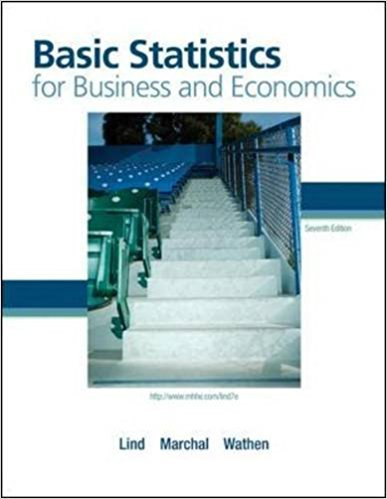×
Get Full Access to Basic Statistics For Business And Economics - 7 Edition - Chapter 6 - Problem 55e
Get Full Access to Basic Statistics For Business And Economics - 7 Edition - Chapter 6 - Problem 55e

×

# The National Aeronautics and Space Administration (NASA)ISBN: 9780077384470 64

## Solution for problem 55E Chapter 6

Basic Statistics for Business and Economics | 7th Edition

• Textbook Solutions
• 2901 Step-by-step solutions solved by professors and subject experts
• Get 24/7 help from StudySoup virtual teaching assistantsBasic Statistics for Business and Economics | 7th Edition

4 5 1 278 Reviews
28
5
Problem 55E

Problem 55E

The National Aeronautics and Space Administration (NASA) has experienced two disasters. The Challenger exploded over the Atlantic Ocean in 1986 and the Columbia exploded over East Texas in 2003. There have been a total of 123 space missions. Assume failures continue to occur at the same rate and consider the next 23 missions. What is the probability of exactly two failures? What is the probability of no failures?

Step-by-Step Solution:

Solution :

Step 1 of 1:

We assume failures continue to occur at the same rate and consider the next 23 missions.

So n=23.

The Poisson distribution formula is

P(X=x) =Where,is the mean number of occurrences in a particular interval.

e is the constant.

x is the number of occurrences.

P(x) is the probability  for a specified the value of x.

Our goal is:

We need to find the probability of exactly two failures and the probability of no failures.

Then the mean of poisson distribution is.

We know that n=23 andis the probability of failure.= 23= 23== 0.4070

Then the poisson formula is

P(X=x) =The probability of exactly two failures is

P(X=2) =P(X=2) =P(X=2) =P(X=2) = 0.0551

Therefore, the probability of exactly two failures is 0.0551.

Then the poisson formula is

P(X=x) =The probability of no failures is

P(X=0) =P(X=0) = 0.6656

Therefore, the probability of no failures is 0.6656.

Step 2 of 1

##### ISBN: 9780077384470

Unlock Textbook Solution